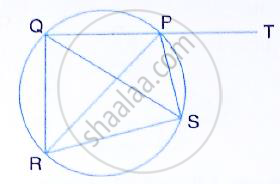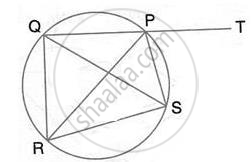Share

In the Given Figure, Sp is Bisector of ∠Rpt and Pqrs is a Cyclic Quadrilateral. Prove that Sq = Sr . - ICSE Class 10 - Mathematics

Question

In the given figure, SP is bisector of ∠RPT and PQRS is a cyclic quadrilateral. Prove that SQ = SR .SolutionPQRS is a cyclic quadrilateral

∠QRS +∠QPS = 180° …………(i)
(pair of opposite angles in a cyclic quadrilateral are supplementary)

Also, QPS +∠SPT = 180°     ……(ii)
(Straight line QPT)

From (i) and (ii)

∠QRS = ∠SPT              ………. (iii)

Also, ∠RQS = ∠RPS              ……(iv)
(Angle subtended by the same chord on the circle are equal)

And ∠RPS = ∠SPT (PS bisects ∠RPT )  …… (v)
From (iii), (iv) and (v)
∠QRS = ∠RQS
⇒ SQ = SR

Is there an error in this question or solution?
Solution In the Given Figure, Sp is Bisector of ∠Rpt and Pqrs is a Cyclic Quadrilateral. Prove that Sq = Sr . Concept: Cyclic Properties.
S